# NCERT Solutions for Chapter 6 Electromagnetic Induction

Question 1.

Predict the direction of induced current in the situations described by the following Fig. 2(a) to (f).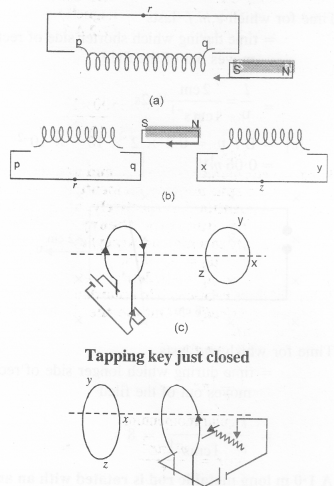(a) By Lenz’s law, the face of the coil towards the south pole of the magnet opposes the south pole. So this face should behave as the south pole. Hence the current flows along qrpq.

(b) Similar to the above reason the current flows along yzxy and along prqp.

(c) When the coil is energised, with a cell the increasing current produces an inverse current in the nearby coil along yzxy.

(d) Similar to the above reason the current flows along zyvz.

(e) By Lenz’s law current is along xryx.

(f) Field lines being along the plane of the loop, there is no induced current.

Question 2.

Use Lenz’s law to determine the direction of induced current in the situations described by Figure:

(a) A wire of irregular shape turning into a circular shape;

(b) A circular loop being deformed into a narrow straight wire.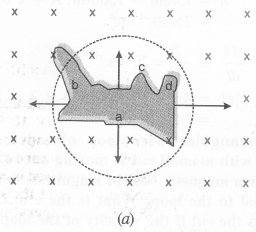When a wire of irregular shape turns into a circular (loop the magnetic flux linked with the loop increases due to an increase in area) The irregular shape. The induced e.m.f. will cause current to flow in such a direction, so that the wire forming the loop is pulled inward from all sides. It requires that the current should flow.

By applying Lenz’s Law, it follows that the current will flow in the direction a, d, c, b, a.

Question 3.

A long solenoid with 15 turns per cm has a small loop of area 2.0 cm2 placed inside the solenoid normal to its axis. If the current carried by the solenoid changes steadily from 2.0 A to 4.0 A in 0.1s, what is the induced e.m.f. in the loop while the current is changing?Question 4.

A rectangular wire loop of sides 8 cm and 2 cm with a small cut ¡s moving out of a region of the uniform magnetic field of magnitude O.3 T directed normal to the mop. What ¡s the e.m.f. developed across the cut if the velocity of the loop is 1 cm s-2 in a direction normal to the (a) longer side, (b) the shorter side of the loop? For how long does the induced voltage last in each case?

e = BlυQuestion 5.

A 1.0 m long metallic rod is rotated with an angular frequency of 400 rad s_1 about an axis normal to the rod passing through its one end. The other end of the rod is in contact with a circular metallic ring. A constant and uniform magnetic field of 0.5 T parallel to the axis exists everywhere. Calculate the e.m.f. developed between the center and the ring.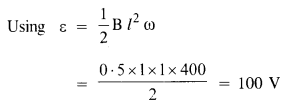Question 6.

A circular coil of radius 8.0 cm and 20 turns rotates about its vertical diameter with an angular speed of 500 rad s_1 a uniform horizontal magnetic field of magnitude 3.0 x 10-2 T. Obtain the maximum and average emf induced in the coil. If the coil forms a closed loop of resistance 10 Ω, calculate the maximum value of current in the coil. Calculate the average power loss due to Joule heating. Where does this power come from?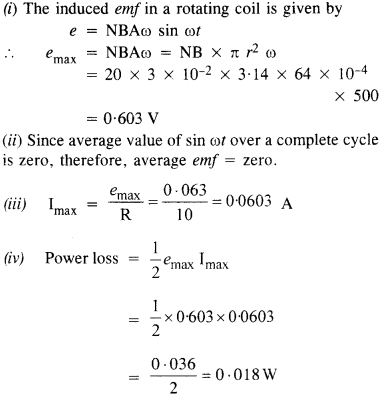(v) Source of power loss is the external rotor which provides the necessary torque to rotate the coil.

Question 7.

A horizontal straight wire 10 m long extending from east to west is falling with a speed of 5.0 ms-1, at right angles to the horizontal component of the earth’s magnetic field, 0.30 x 10-4 Wb m-2.

(a) What is the instantaneous value of the e.m.f. induced in the wire?

(b) What is the direction of the e.m.f.?

(c) Which end of the wire is at the higher electrical potential?(b) Using Fleming’s Right-hand rule, the direction of induced e.m.f. is from West to East.

(c) Since the rod will act as a source, the Western end will be at a higher electrical potential.

Question 8.

Current in a circuit falls from 5.0 A to 0.0 As. If an average emf of 200 V is induced, give an estimate of the self-inductance of the circuit.Question 9.

A pair of adjacent coils has a mutual inductance of 1.5 H. If the current in one coil changes from 0 to 20 A in 0.5 s, what is the change of flux linkage with the other coil?

B = M.dl = 1.5 x (20 – 0) = 30 Wb

Question 10.

A jet plane is travelling towards the west at a speed of 1800 km/h. What is the voltage difference developed between the ends of the wing having a span of 25 m, if the Earth’s magnetic field at the location has a magnitude of 5 x 10-4 T and the dip angle is 30°?Question 11.

Suppose the loop in Exercise 6.4 is stationary but the current feeding the electromagnet that produces the magnetic field is gradually reduced so that the field decreases from its initial value of 0.3 T at the rate of 0.02 T s-1. If the cut is joined and the loop has a resistance of 1.6 Ω, how much power is dissipated by the loop as heat? What is the source of this power?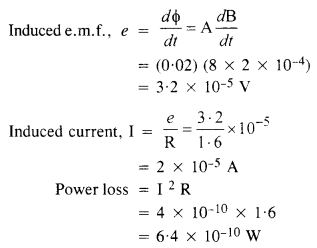The Source of this power is the external agency which brings a change in a magnetic field.

Question 12.

A square loop of side 12 cm with its sides parallel to X and Y axes is moved with a velocity of 8 cm s_1 in the positive x-direction in an environment containing a magnetic field in the positive z-direction. The field is neither uniform in space nor constant in time. It has a gradient of 10-3 T cm-1 along the negative x-direction (that is it increases by 10-3 T cm-1 as one moves in the negative x-direction), and it is decreasing in time at the rate of 10-3 T s_1. Determine the direction and magnitude of the induced current in the loop if its resistance is 4.50 mΩ.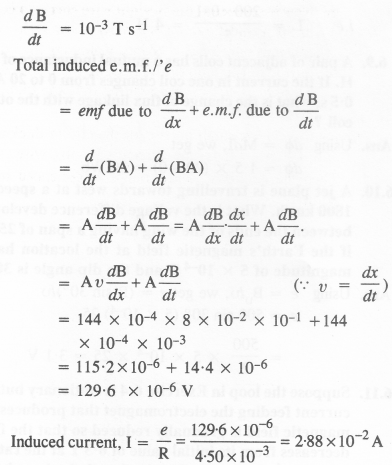The direction of the induced current is such that it increases the magnetic flux linking with the loop in positive 2-direction.

Question 13.

It is desired to measure the magnitude of the field between the poles of a powerful loudspeaker magnet. A small flat search coil of area 2 cm2 with 25 closely wound turns, is positioned normal to the field direction, and then quickly snatched out of the field region. Equivalently, one can give it a quick 90° turn to bring its plane parallel to the field direction. The total charge flew in the coil (measured by a ballistic galvanometer connected to the coil) is 7.5 mC. The combined resistance of the coil and the galvanometer is 0.50 Ω. Estimate the field strength of the magnet.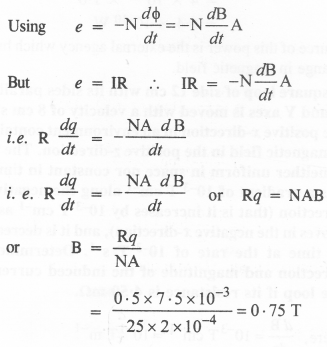Question 14.

The figure shows a metal rod PQ resting on the rails AB and positioned between the poles of a permanent magnet. The rails, the rod, and the magnetic field are in three mutually perpendicular directions. A galvanometer G connects the rails through a switch K. Length of the rod = 15 cm, B = 0.50 T, resistance of the closed-loop containing the rod = 9.0 mΩ. Assume the field to be uniform.(a) Suppose K is open and the rod is moved with a speed of 12 cm s-1 in the direction shown. Give the polarity and magnitude of the induced emf.

(b) Is there an excess charge built up at the ends of the rods when K is open? What if K is closed?

(c) With K open and the rod moving uniformly, there is no net force on the electrons in the rod PQ even though they do experience a magnetic force due to the motion of the rod. Explain.

(d) What is the retarding force on the rod when K is closed?

(e) How much power is required (by an external agent) to keep the rod moving at the same speed (= 12 cm s_1) when K is closed? How much power is required when K is open?

(f) How much power is dissipated as heat in the closed-circuit? What is the source of this power?

(g) What is the induced emf in the moving rod if the magnetic field is parallel to the rails instead of being perpendicular?

Here, B = 0.50 T; 1 = 15 cm = 15 x 10-2 m;

R = 9.0m Q = 9.0 x 103 fl

(a) Now, e = Bvl

Here, V = 12 cm s-1 = 12 x 10-2 ms-1

∴ e = 0.50 x 12 x 10-2x 15 x 10 2 = 9 x 10-3V

If q is a charge on as electron, then the electrons in the rod will experience magnetic Lorentz force −q[v⃗ +B⃗ ] P. Q. Hence, the end P of the rod will become positive and the end Q will become negative.

(b) When the switch K is open, the electron collects at the end Q. Therefore, excess change is built up at the end Q. However, when the switch K is closed, the accumulated charge at the end Q flows through the circuit,

(c) The magnetic Lorentz force on the electron is cancelled by the electronic force acting on it due to the electronic field set up across the two ends due to the accumulation of positive and negative charges at the ends P and Q respectively.

(d) Retarding force, F = BIl =B eRQuestion 15.

An air-cored solenoid with length 30 cm, area of cross-section 25 cm2 and number of turns 500, carries a current of 2.5 A. The current is suddenly switched off in a brief time of 10-3 s. How much is the average back emf induced across the ends of the open switch in the circuit ? Ignore the variation in magnetic field near the ends of the solenoid.Question 16.

(a) Obtain an expression for the mutual inductance between a long straight wire and a square loop of side a as shown in Figure(b) Now assume that the straight wire carries a current of 50 A and the loop is moved to the right with a constant velocity, υ = 10 m/s. Calculate the induced e.m.f. in the loop at the instant when x = 0.2 m. Take a = 0.1 m and assume that the loop has a large resistance.

(a) Consider a small portion of the coil of thickness dt at a distance t from the current-carrying wire. Then the magnetic field strength experienced by this portion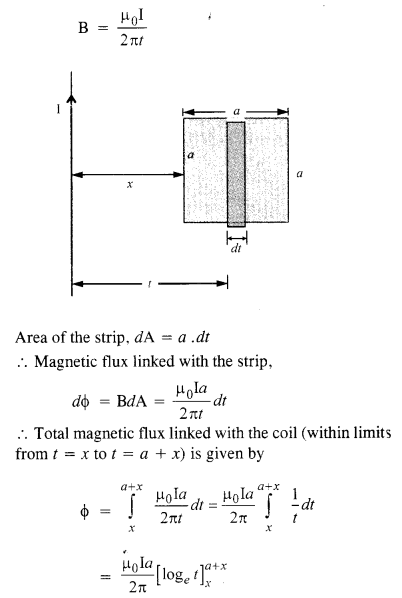Question 17.

A line charge X per unit length is lodged uniformly onto the rim of a wheel of mass M and radius R. The wheel has light non-conducting spokes and is free to rotate without friction about its axis (Figure.) A uniform magnetic field extends over a circular region within the rim. It is given byWhat is the angular velocity of the wheel after the field is suddenly switched off?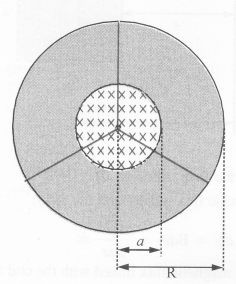Change in magnetic field is given by,